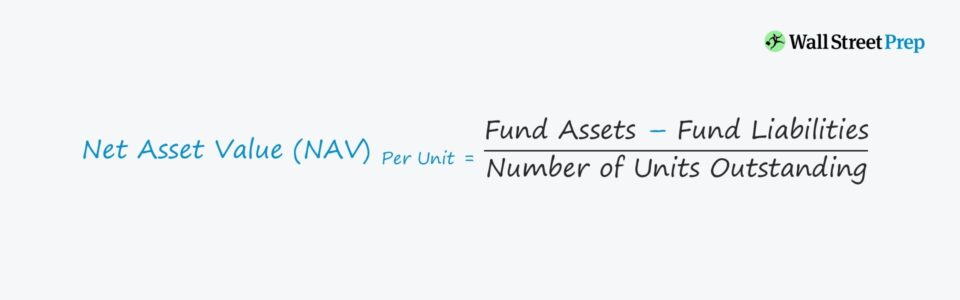# Net Asset Value (NAV)

Understand the Net Asset Value (NAV) Concept• What is the definition of net asset value (NAV)?
• What is the formula for calculating net asset value (NAV)?
• How is NAV used to measure mutual fund performance?
• For investments funds, at what time on a trading day is the NAV calculated?

## Net Asset Value (NAV) and Mutual Funds

The net asset value (NAV) commonly appears in the context of mutual funds, as the metric serves as the basis for setting the mutual fund share price.

NAV on a per-unit basis represents the price at which units (i.e. ownership shares) in the mutual fund can be purchased or redeemed, which is typically done at the end of each trading day.

A mutual fund’s NAV is a function of the market value of all securities held in its portfolio.

• If Portfolio Value Increases ➝ NAV Increases
• If Portfolio Value Decreases ➝ NAV Declines

However, just as in the case of valuing individual public equities, the past performance of a mutual fund is not reflective of future performance.

Fund investors must ask, “Will the mutual fund’s strategy continue to perform well into the future?”

In addition, the mutual fund’s objective, risk/return profile, and time horizon must align with the criteria of the investor, rather than solely evaluating funds based on their NAV.

## Net Asset Value (NAV) Formula

The NAV of a mutual fund is calculated by subtracting the total liabilities from its total assets.

Since NAV is typically expressed on a unit price basis, i.e. per share, NAV must be divided by the total number of units outstanding.

• Net Asset Value (NAV) = Fund Assets – Fund Liabilities
• Net Asset Value (NAV) Per Unit = (Fund Assets – Fund Liabilities) ÷ Number of Units Outstanding

For example, if a mutual fund’s total holdings are valued at \$100 million with liabilities of \$20 million, the fund’s NAV is equal to \$80 million.

• NAV = \$100m – \$20m = \$80m

Now that we’ve subtracted what the fund owes (liabilities) from the value of what the fund owns (assets), the next step is to divide by the total number of units outstanding.

If we assume the mutual fund has 2 million units outstanding, the NAV per share comes out to \$40.00.

• NAV Per Share = \$80 million ÷ 2 million

Oftentimes, there is a disconnect between the net asset value (NAV) and market price.

For example, the NAV of an exchange-traded fund (ETF) is based on its underlying securities, whereas the ETF market price is based on supply/demand in the market.

So far, we’ve discussed net asset value (NAV) in the context of mutual funds, but another use-case of NAV is in real-estate investing – e.g. real estate investment trusts (REITs).

Here, NAV is equal to the fair market value (FMV) of real estate assets minus any outstanding debt, fixed costs, and capital expenditures (CapEx).

### NAV REIT Valuation Model Steps

1. Value Net Operating Income (NOI) Generating Assets in Real Estate Portfolio
2. Value Side-Income Streams – e.g. Ancillary Income, Management Fees, Joint Venture (JV) Income
3. Reduce NAV by Required Costs (e.g. Overhead) and Future Expected Capital Expenditures (CapEx)
4. Add “Non-Operating” REIT Assets – e.g. Cash, Construction-in-Progress
5. Subtract Outstanding Debt Obligation and Non-Equity Claims

After the final step, the end result is the NAV-derived equity value, which can be divided by shares outstanding to compare to its market share price.

The book values of U.S. REITs are not marked up to their fair market value (FMV) – but the NAV model adjusts the balance sheet of a REIT to reflect the FMV of assets with capitalization rate (“cap rate”) assumptions.

NAV is the preferred valuation approach in real estate transactions.Globally Recognized Certification Program

#### Get the Fixed Income Markets Certification (FIMC©)

Wall Street Prep's globally recognized certification program prepares trainees with the skills they need to succeed as a Fixed Income Trader on either the Buy Side or Sell Side.Inline FeedbacksGet the WSP Fixed Income Markets Certification (FIMC©)

Wall Street Prep’s globally recognized certification program prepares trainees with the skills they need to succeed as a Fixed Income Trader on either the Buy Side or Sell Side.

X

The Wall Street Prep Quicklesson Series

7 Free Financial Modeling Lessons

Get instant access to video lessons taught by experienced investment bankers. Learn financial statement modeling, DCF, M&A, LBO, Comps and Excel shortcuts.